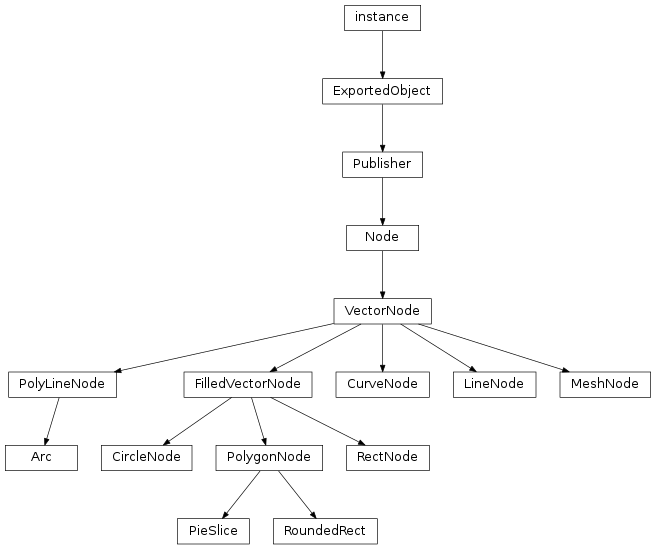# Vector Nodes¶class `libavg.avg.``CircleNode`([r=1, texcoord1=0, texcoord2=1])

A circle. The reference point for a circle is its center.

`r`

The radius of the circle in pixels.

`texcoord1`
`texcoord2`

floats used as u texture coordinates for the circle border. The coordinates wrap around the circle once.

class `libavg.avg.``CurveNode`([pos1, pos2, pos3, pos4, texcoord1, texcoord2])

A cubic bezier curve (http://en.wikipedia.org/wiki/Bezier_curve). `pos1` and `pos4` are the two endpoints of the curve. `pos2` and `pos3` are control points.

`getPtOnCurve`(t) → pos

Returns a point on the curve. Which point is determined by the value of t. If `t=0`, returns `pos1`. `t=1` returns `pos4`, and values in between return the points in between.

`length`

Returns an approximation of the length of the curve (ro).

`pos1`
`pos2`
`pos3`
`pos4`
`texcoord1`
`texcoord2`

floats that signify the u axis texture coordinates.

class `libavg.avg.``FilledVectorNode`([filltexhref, fillopacity=0, fillcolor="FFFFFF", filltexcoord1=Point2D(0, 0), filltexcoord2=Point2D(1, 1)])

Base class for vector nodes which have a filled area and a border. The area can be filled either with a solid color (`fillcolor`) or with a texture loaded from a file (`filltexhref`), or taken from a bitmap object (`setFillBitmap()`).

`fillcolor`
`fillopacity`
`filltexcoord1`
`filltexcoord2`
`filltexhref`

An image file to use as a texture for the area of the node.

`setFillBitmap`(bitmap)

Sets a bitmap to use as a fill texture. Sets `filltexhref` to an empty string.

class `libavg.avg.``LineNode`([pos1, pos2, texcoord1, texcoord2])

A line. `pos1` and `pos2` are the two endpoints of the line.

`pos1`
`pos2`
`texcoord1`
`texcoord2`

floats that signify the u axis texture coordinates.

class `libavg.avg.``MeshNode`([vertexcoords, texcoords, triangles])

This is a generalized mesh of textured triangles. See https://www.libavg.de/wiki/ProgrammersGuide/MeshNode for an example.

`texcoords`
`triangles`
`vertexcoords`
class `libavg.avg.``PolygonNode`([linejoin="bevel", pos, texcoords])

A closed figure bounded by a number of line segments, optionally filled. Filled polygons may not be self-intersecting.

`linejoin`

The method by which line segments are joined together. Valid values are `bevel` and `miter`.

`pos`

A sequence (`list` or `tuple`) of pixel positions.

`texcoords`

A sequence of float texture coordinates along the u axis of the texture, used for the polygon border. It can contain either one coordinate per entry in pos (plus an additional one to close the polygon) or two coordinates that are interpolated to wrap around the `PolygonNode` once.

class `libavg.avg.``PolyLineNode`([linejoin="bevel", pos, texcoords])

A figure similar to a `PolygonNode`, but not closed and never filled.

`linejoin`

The method by which line segments are joined together. Valid values are `bevel` and `miter`.

`pos`

A sequence (`list` or `tuple`) of pixel positions.

`texcoords`

A sequence of float texture coordinates along the u axis of the texture. It can contain either one coordinate per entry in pos or two coordinates, one for either end of the `PolyLine`.

class `libavg.avg.``RectNode`([pos, size, angle])

A rectangle that can be filled.

`angle`

The angle that the rectangle is rotated to in radians. 0 is unchanged, 3.14 is upside-down. The rectangle is rotated around its center.

`pos`

The position of the top left corner of the rectangle.

`size`
`texcoords`

A sequence of `5` texture coordinates for the border of the rectangle, which wrap around the node.

class `libavg.avg.``VectorNode`([color="FFFFFF", strokewidth=1, texhref, blendmode="blend"])

Base class for all nodes that draw geometrical primitives. All vector nodes support configurable stroke width. Strokes can be filled either with a solid color (`color`) or with a texture loaded from a file (`texhref`) or taken from a bitmap object (`setBitmap()`).

When using textured `VectorNodes`, texture coordinates can be set. Generally, texture bitmaps are mapped to coordinates `(u,v) = (0,0)-(1,1)`, and the texture is repeated for coordinates outside this range. The `u` texture coordinate increases along the stroke, while the `v` coordinate increases perpendicular to it.

`blendmode`

The method of compositing the node with the nodes under it. Valid values are `blend`, `add`, `min` and `max`. For `min` and `max` blend modes, opacity is ignored.

`color`

The `Color` of the strokes.

`strokewidth`

The width of the strokes in the vector. For lines, this is the line width. For rectangles, it is the width of the outline, etc.

`texhref`

An image file to use as a texture for the node.

`setBitmap`(bitmap)

Sets a bitmap to use as a texture. Sets `texhref` to an empty string.

class `libavg.geom.``Arc`(radius, startangle, endangle[, pos=(0, 0)])

An unfilled arc (incomplete circle) from `startangle` to `endangle`. `pos` is the center of the circle.

`endangle`
`pos`
`radius`
`startangle`
class `libavg.geom.``PieSlice`(radius, startangle, endangle[, pos=(0, 0)])

An arc (incomplete circle) from `startangle` to `endangle` connected to the center of the circle. `pos` is the center of the circle. A `PieSlice` can be filled.

`endangle`
`pos`
`radius`
`startangle`
class `libavg.geom.``RoundedRect`(size, radius, pos)

A rectangle with rounded corners. `radius` is the corner radius.

`pos`
`radius`
`size`